# Math > Year 2

## Describing Direction

When we are giving directions we use keywords such as: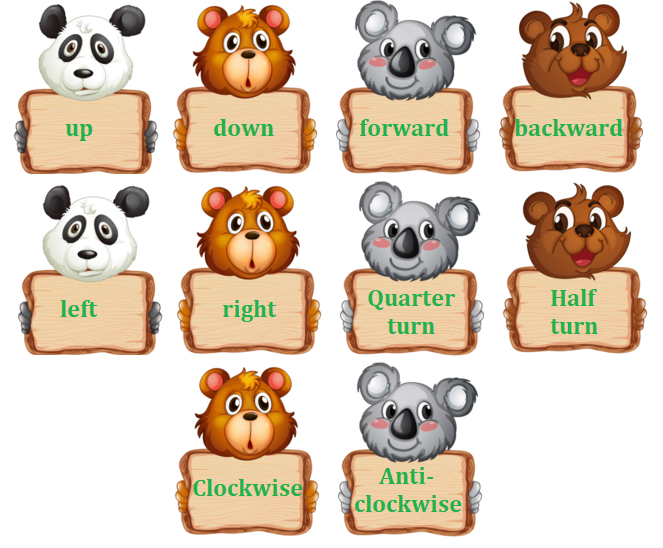Clockwise and anticlockwise direction: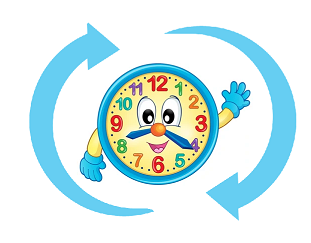The direction in which the hands of a clock move is called the clockwise direction.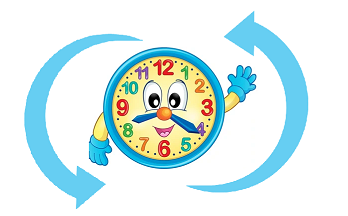The opposite direction to the direction in which the hands of a clock move is called the anticlockwise direction.

Forward and Backward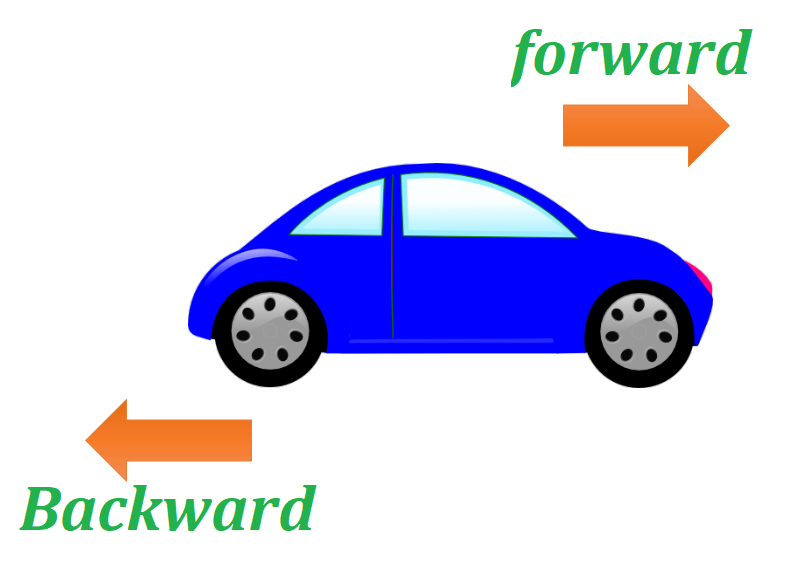If a car moves towards the front side then it is called moving forwards.
If a car moves towards the back side then it is called moving backwards.

Let’s learn about different types of turns

Quarter Turn

A quarter turn is equal to a right angle and means turning left or right.

Example: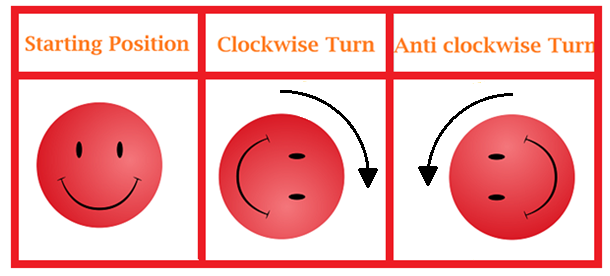Half Turn

Two quarter turns make a half turn. A half-turn is turning halfway to face the opposite direction.

Example: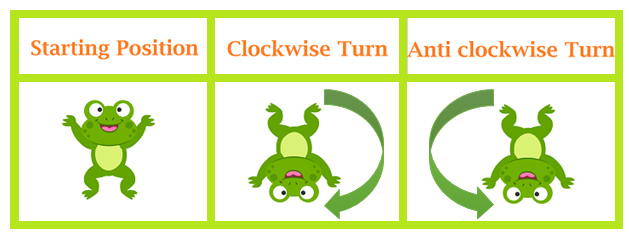Three Quarter Turn

A quarter turn thrice makes a three-quarter turn.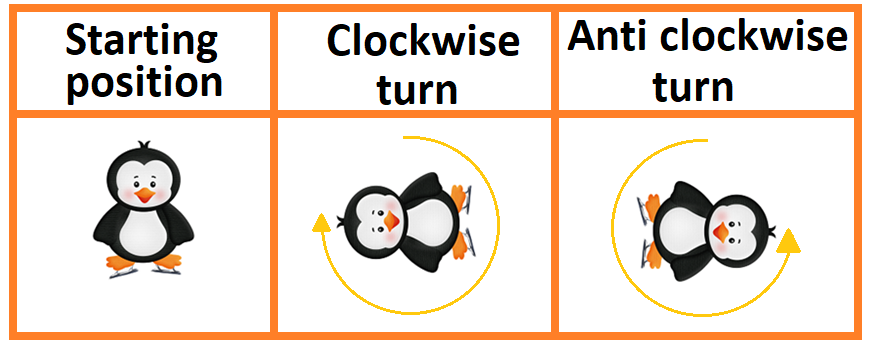A full turn is turning all the way around and ending up in the same place that you started in.

Let’s take a look at one more example to describe direction: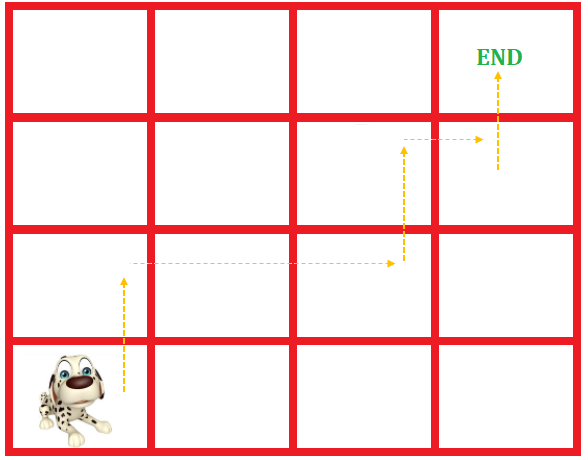The dog has followed the directions to reach the endpoint.

The dog has moved one square up.

Then two squares forward towards the right.

Then one square up.

The one square towards the right.

Then one square up.

Let’s try a question:

Question: The given teddy bear has moved _________________.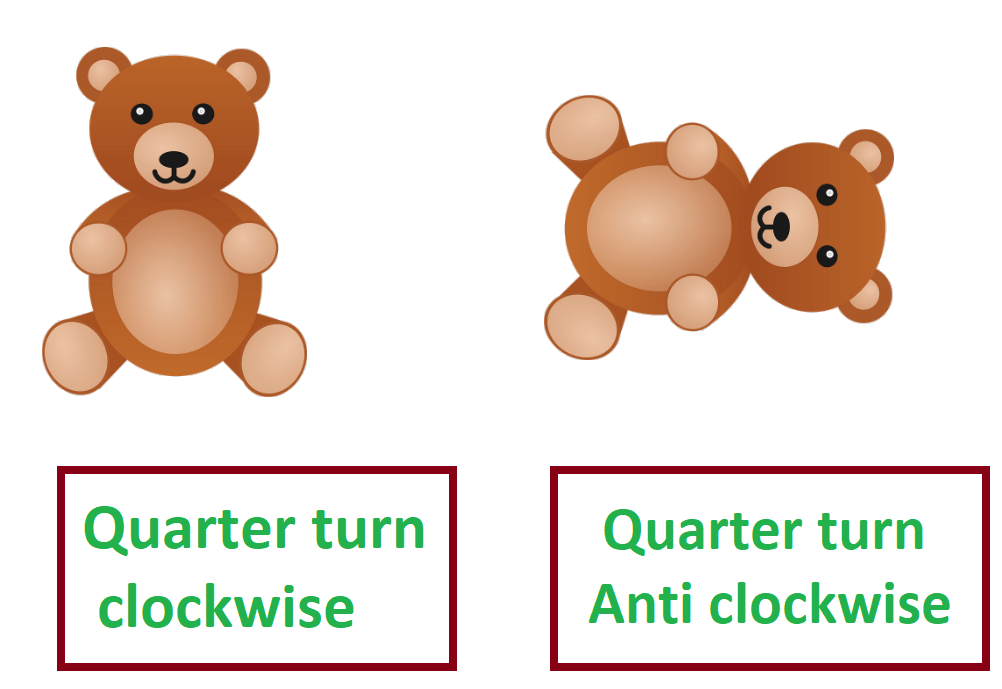Explanation: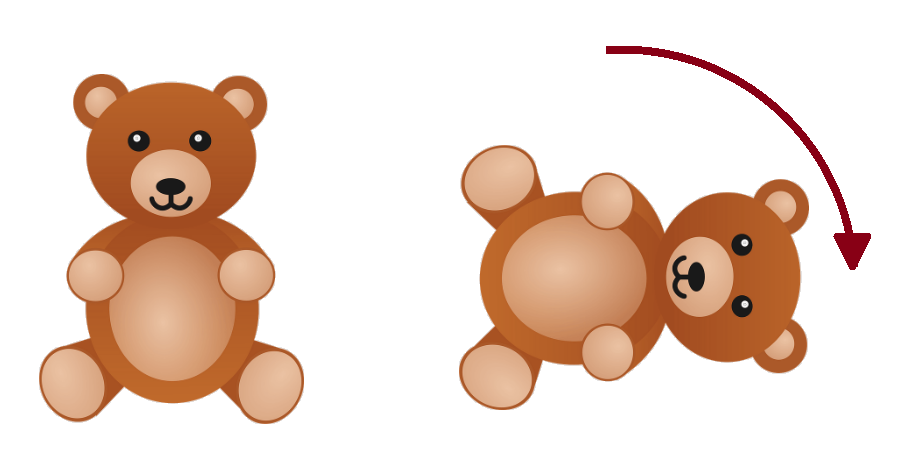The teddy bear has moved a quarter turn clockwise.

//